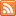# Content Tagged “Probability”Probability is the “machinery” that allows us to draw conclusions about the population based on the data collected in the sample.

## Unit 3B: Random Variables

Published: Aug 27th, 2012

Introduction Random Variables Types of Random Variables CO-6: Apply basic concepts of probability, random variation, and commonly used statistical probability distributions. Video: Unit 3B Random Variables (10:00) Introduction In the remaining sections in […]## Unit 1: Exploratory Data Analysis

Published: Jul 30th, 2012

The Big Picture Exploratory Data Analysis Important Features of Exploratory Data Analysis Examining Distributions CO-1: Describe the roles biostatistics serves in the discipline of public health. CO-6: Apply basic concepts of […]## The Big Picture

Published: Jul 30th, 2012

CO-1: Describe the roles biostatistics serves in the discipline of public health. Throughout the course, we will add to our understanding of the definitions, concepts, and processes which are introduced here. […]## Conditional Probability and Independence

Published: Jul 28th, 2012

Independent Events Multiplication Rule for Independent Events (Rule Six) Conditional Probability (Rule Seven) Independent Events (Part 2) General Multiplication Rule (Rule Eight) Let’s Summarize CO-6: Apply basic concepts of probability, random […]## Basic Probability Rules

Published: Jul 28th, 2012

Introduction Rules of Probability Probability Rule One (For any event A, 0 ≤ P(A) ≤ 1) Probability Rule Two (The sum of the probabilities of all possible outcomes is 1) Probability Rule […]## Introduction to Probability

Published: Jul 28th, 2012

Probability is Not Always Intuitive Need a Laugh? What is Probability? Probability is the Likelihood of Something Happening Notation Determining Probability To Summarize So Far Relative Frequency Let’s Summarize CO-6: Apply […]## Unit 3A: Probability

Published: Jul 28th, 2012

Review of the Big Picture Probability and Inference CO-1: Describe the roles biostatistics serves in the discipline of public health. CO-6: Apply basic concepts of probability, random variation, and commonly used statistical […]